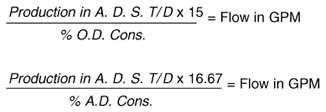### Definition/ConversionFactors

A.D. = Air Dry stock (Contains 10% Water)

O.D. = Oven Dry stock (All Water Removed) Also Called Bone Dry (B.D.)

A.D. = 1.11 x O.D.

A.D. = 1.11 O.D.T/D

O.D. = 0.90 x A.D. T/ D

A.D. Consistency = 1.11 x O.D. Consistency

O.D. Consistency = 0.90 x A.D. Consistency

T/ D or TPD or S. T/D = Short Tons Per Day One Short Ton = 2000 lbs.

M. T/ D = Metric Tons per Day One Metric Ton = 2205 lbs.

A.D.S. T/ D = Air Dry Short Tons/Day

A.D.M. T/D = Alr Dry Metric Tons/Day

S. T/ D = 1.1025 x M. T/ D